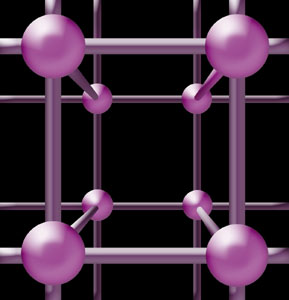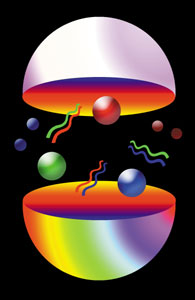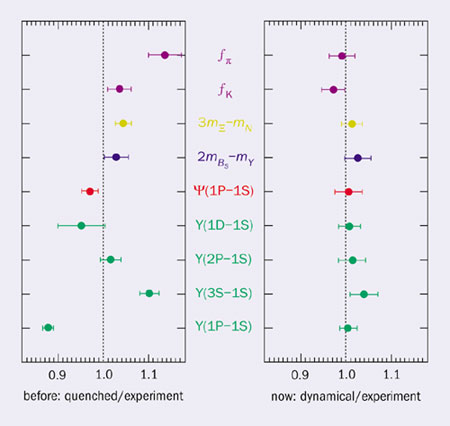# Joining up the dots with the strong force

7 June 2004

A new variation on an old technique is yielding the most precise results so far in lattice calculations of quantum chromodynamics, the theory of strong interactions, as Christine Davies explains.In lattice QCD space-time is approximated by a four-dimensional box of points, similar to a crystal lattice. (Ian McVicar/Glasgow.)

Experimental particle physicists are well used to the fact that many years must elapse between the planning of a big experiment and the analysis of the results. Theorists do not generally have to wait so long for their ideas to bear fruit.

The attempt to solve the theory of the strong force by numerical simulation, however, has been a long-running saga. The technique, called lattice quantum chromodynamics, or more usually lattice QCD, was suggested 30 years ago and first attempted numerically in the late 1970s. Since then particle theorists have tried to monopolize each generation of the world’s fastest supercomputers with their calculations, and battled with improved algorithms and sources of systematic error. Now they are close to a solution at last.

New calculations, which have simulated the most realistic QCD vacuum to date, have shown agreement with experiment for simple hadron masses for the first time at the level of a few percent. This is an important milestone. Only when well known quantities are accurately reproduced can we have faith in the calculation of other quantities that experiment cannot determine. A number of such calculations are eagerly awaited by the particle-physics community as a new era of high-precision calculations in lattice QCD begins.

### Precision testing

Precise lattice QCD calculations are needed as part of the worldwide programme of testing the Standard Model so rigorously that flaws are exposed that will allow us to develop a deeper theory, explaining nature more completely. QCD is a key component of the Standard Model because any experiment aimed at the study of quark interactions must necessarily confront the issue that quarks are confined inside hadrons by the strong interactions of QCD. It is this feature of QCD that makes it hard to tackle theoretically as well. Although perturbation theory works well for QCD when high energies are involved, such as in jet physics, it is not an appropriate tool for physics at the hadronic scale. QCD interactions are so much stronger there that a fully non-perturbative calculation must be done using lattice QCD.

An important example of where lattice QCD calculations are needed is the attempt by experiments at B-factories to test the self-consistency of the Standard Model through the determination of the elements of the Cabibbo-Kobayashi-Maskawa (CKM) matrix. This is now seriously limited by the precision with which QCD effects can be included in theoretical calculations of B-meson mixing and decay rates. Errors of a few percent are needed to match the experimental precision. Another important task is that of the determination of quark masses and the QCD coupling constant, αs. Like the CKM matrix elements, they are fundamental parameters of the Standard Model that are a priori unknown and must be determined from experiment. Because quarks cannot be observed as isolated particles we cannot determine their masses or colour charges directly and theoretical input is required.

In lattice QCD the procedure is straightforward but numerically challenging. We must solve QCD for well known observable quantities, such as hadron masses, as a function of the quark masses and the coupling constant in the QCD Lagrangian. The value of a quark mass is then that which gives a particular hadron mass in agreement with experiment. The scale of QCD, Λ, is likewise determined by the requirement for another hadron mass to have its experimental value, and this is equivalent to determining the coupling constant. Quark masses, particularly those for u, d, s and c, are rather poorly known at present and this hampers a number of phenomenological studies. Precision of a few percent, rather than the current 30%, on the s quark mass would greatly reduce theoretical errors in the CP-violating parameter ε´/ε of kaon physics, for example.

### The space-time lattice

Lattice QCD proceeds by approximating a chunk of the space-time continuum using a four-dimensional box of points, similar to a crystal lattice. The quark and gluon quantum fields then take values only at the lattice points or on the links between them, and the equations of QCD are discretized by replacing derivatives with finite differences. In this way a problem that would be infinitely difficult is reduced to something tractable in principle. We still have to perform a many-dimensional integral over the fields that we have, however, and this is done by an intelligent Monte Carlo process that preferentially generates field configurations of the QCD vacuum that contribute most to the integral.The proton contains three valence quarks of three different colours (red, green and blue), but it also contains dynamical (sea) quarks. These are quark-antiquark pairs that appear and disappear through energy fluctuations in the vacuum. In the past these dynamical quarks presented big problems in lattice calculations of particle masses. (Ian McVicar/Glasgow.)

This part of a lattice QCD calculation increasingly resembles a particle-physics experiment. Collaborations of theorists with access to a powerful supercomputer generate these configurations and store them for the second phase, which resembles the data analysis that experimentalists perform. In this phase hadron correlation functions are calculated on the configurations and fits are performed to determine hadron masses and properties. Many hundreds of configurations are typically needed to reduce the statistical error from the Monte Carlo to less than 1%.

Lattice QCD results are calculated for a lattice with a finite volume and a finite lattice spacing, but we want them to be relevant to the infinite volume and zero lattice spacing of the real world. For the accuracy we need we must understand how the results depend on the volume and lattice spacing, and reduce the systematic error from this dependence below the 1% level. The dependence on volume of most results falls very rapidly for large-enough volumes, so lattices 2.5 fm across or larger are thought to be sufficient for calculations at present. The dependence on lattice spacing is more difficult to remove and this was the subject of a great deal of work throughout the 1990s. The development of higher order, “improved” discretizations of QCD has allowed calculations to be performed that give answers close to continuum QCD, with values for the lattice spacing of around 0.1 fm. These are feasible on current supercomputers. With unimproved discretizations we would need to work with lattice spacing values 10 times smaller to achieve the same systematic error. This would cost, even naively, a factor of 10,000 in computer time, and in practice much more.

One key problem remained at the end of the 1990s. This was the huge computational cost of including the effect of dynamical (sea) quarks: u, d and s quark-antiquark pairs that appear and disappear through energy fluctuations in the vacuum (c, b and t quarks are too heavy to have any significant effect). The anticommuting nature of quarks, as fermions, means that their fields cannot be represented directly on the computer as the gluon fields are. Instead the quark fields are “integrated out” and the effect of dynamical quarks then appears as the determinant of the enormous quark interaction matrix that connects quark fields at different points. The dimension of the matrix is the volume of the lattice times 12 for quark colour and spin, typically 107.

### Honing techniques

The “quenched approximation”, used extensively in the past, misses out the quark determinant entirely, and this is clearly inadequate for precision results. However, it has been a useful testing ground for theorists to hone their analysis techniques. More recently, dynamical quarks have been included, but often these are only u and d quarks with masses many times heavier than the very light values in the real world because the cost of including the quark determinant rises as the quark mass falls. A figure of merit for modern lattice calculations is how light the u and d quark masses (almost always taken to be the same) are in terms of the s quark mass (ms). Given a range of u/d masses below ms/2, it should be possible to extrapolate down to physical results using chiral perturbation theory. Early simulations with dynamical quarks struggled to reach ms/2 from above and so it was hard to distinguish results from the quenched approximation, although encouraging signs of the effects of dynamical quarks were seen.

Recent simulations by the MILC collaboration, however, have managed to include u, d and s dynamical quarks with four different values of the u/d quark mass below ms/2 and going as low as ms/8. This has allowed well controlled extrapolations to find the physical u/d quark mass where the (isospin averaged) pion mass agrees with experiment. Two different values of the lattice spacing have been simulated to check discretization errors and two different volumes (2.5 and 3.5 fm across) to check finite volume errors.

These results have been made possible by a new formulation of quarks in lattice QCD called the improved staggered formulation. The staggered formulation is an old one and has always been very quick to simulate. It uses the most naive discretization of the Dirac action possible on the lattice and the quark spin degree of freedom is then in fact redundant, immediately reducing the dimension of the quark interaction matrix by a factor of four. The discretization errors were originally very large with this formalism, however, and it is the realization that these can be removed using the improvement methodology discussed above that has enabled the improved staggered formalism to be a viable one for precision calculations.Fig. 1. New results (right) from lattice QCD calculations that include the effects of dynamical quarks come much closer to the experimental values for various quantities related to the hadron spectrum. On the left, similar ratios for a calculation that ignores dynamical quarks are plotted for comparison.

One caveat remains. Because of the space-time lattice and the notorious “doubling” problem, the staggered formalism actually contains four copies (called “tastes”) of each quark. This four-fold over counting is removed by taking the fourth root of the determinant of the quark matrix when generating configurations that include dynamical quarks. Some theorists object that the fourth root, despite being correct in perturbation theory, may introduce errors in a non-perturbative context. Extensive testing is required to be sure that we do have real QCD, but this is exactly the testing of lattice QCD that is necessary anyway to assure ourselves that precision calculations are possible. So far the formalism has passed with flying colours.

Figure 1 shows the results of an analysis using the MILC configurations by the MILC, HPQCD, UKQCD and Fermilab collaborations. Nine different quantities are plotted, covering the entire range of the hadron spectrum from light hadrons represented by the π and K decay constants (related to the leptonic decay rate) all the way to the heavy hadrons represented by orbital and radial excitation energies in the ϒ system. Light baryons, B-mesons and charmonium are also included. These quantities have been chosen to be “gold-plated” – that is, masses or decay constants of hadrons that are stable in QCD and therefore well defined both theoretically and experimentally. Lattice QCD calculations of these must work if lattice QCD is to be trusted at all. The quark masses and QCD scale have been fixed (as they must be) using other gold-plated hadron masses that do not appear in the plot. These are the masses of the π, K, Ds and ϒ, and the splitting between the ϒ´ and the ϒ. In the figure on the left-hand side are plotted results in the quenched approximation, in which dynamical quarks are ignored. Some quantities disagree with experiment by 10% and there is internal inconsistency in the sense that quantities can be shifted in or out of agreement with experiment by changing the hadrons used to fix the parameters of QCD. On the right are the new results, which include u, d and s dynamical quarks. Now all the quantities agree with experiment simultaneously, as they must if we are simulating real QCD, and this is tested with a precision of a few percent.

### Calculating with confidence

This is a major advance. Now calculations of other quantities can be carried out, knowing that the correct answer in QCD should be obtained. For example, calculations of leptonic and semileptonic decay rates for B and D mesons and B-mixing rates are in progress. Checks of the D results, providing confidence in the B results for the B-factories, will be possible against measurements from the CLEO-c experiment at Cornell. Masses of hadrons that are unstable or close to threshold will be more difficult to calculate with high precision, but many of these, such as glueballs and pentaquarks, are very interesting states. Other quark formulations that escape the doubling problem but are much more costly to simulate will provide important checks once the necessary computational resources are available. Supercomputing for lattice QCD is just entering the teraflops era, and it promises to be a very productive one in which precision calculations are possible at last.